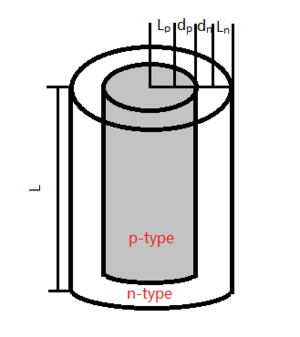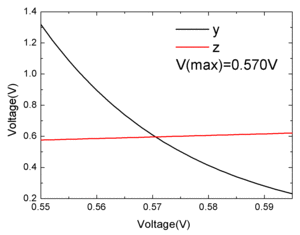Nanowire Solar Cell

Haotian Wang December 17, 2012

Submitted as coursework for PH240, Stanford University, Fall 2012Fig. 1: Schematic of the nanowire solar cell.

China has become the second biggest country in energy consumption and the demand is still increasing. Developing the renewable energies should be an urgent topic in China as well as the whole world with the consideration of the energy crisis and the global environmental problems such as the Green House Effects and Climate Change. Solar energy has been the most attractive renewable energy source, and how to effectively transform it to the energies available to human beings has been discussed for many years. With the development of nano materials science, nanowire solar cells have shown its potential to be efficient and cheap. [1-4] In this paper I will explain the advantages and show some good performance of nanowire solar cells, which should be produced in a large scale in China to replace some energy sources and protect the environment.

The Model and Theory

In order to lower the cost of thin film solar cells, people use silicon with high level of impurities and density of defects, which results in low minority-carrier diffusion lengths.  According to the equation I = I0e - αz. We also want to increase the thickness of p-n junction to absorb as much light as we can and improve its efficiency. These are contradictory because the carrier collection is limited by the short minority-carrier diffusion length in thick solar cells. However, a nanowore solar cell with a p-n junction in the radial direction can separate light absorbtion and carrier extraction, which is shown in Fig. 1. Each individual p-n junction nanowire in the cell could be long in the direction of incident light, allowing for optimal light absorption, but thin in another dimension, thereby allowing for effective carrier collection.

To simplify the analysis, the carrier transport was taken to be purely radial. We assume the minority-carrier diffusion length in n and p region is Ln and Lp respectively. We also set that the depletion region of each side is dn and dp. In order to take all the minorities, we can imagine a nanowire with the radius equals to Ln + Lp + dn + dp = r. The cross-section area of the solar cell is S=πr2. The length of the nanowire is also an important parameter which influence the absorption of incident light. We set the absorption coefficient as α. To get 95% of the light we choose the length of the nanowire to be 3/α.

We assume the doping concentration in n and p type silicon is the same, with NA = ND = 1018 cm-3. With the doping information, we can firstly settle down all the other parameters such as Ln, Lp, and so on. From some physics classes we know that the size of the depletion region should be:

W = dn + dp = [2εSVbi(NA + ND)/eNAND]1/2 = (4εSVbi/eNA)1/2Fig. 2: The numerical calculation of Vmax.

and

Vbi = kBTln(NAND/ni2)/e

The left parameters are Ln and Lp, which have the expression as Ln = (τnDn)1/2 and Lp = (τpDp)1/2. From Einstein Relation we know that

Dn,p = kBn,p/e

The question is how to calculate the lifetime of minority carriers in quasineutral in each type of region, τn and τp. Learnt from other semiconductor materials, we get to know that with highly doped semiconductors τn = 1/σnNrυth, and τp = 1/σpNrυth, where σ n and σp are the cross sections for electron and hole capture, respectively, Nr is the density of recombination centers which can be simply assume as the density of doping, and υth is the thermal velocity. To the first order, σn = σp, so that τn = τp. With all the equations described above, we can now calculate all the parameters about this nanowire under room temperature: 

Vbi = 0.02586 V × ln(1036/6.83 × 1019) = 0.963 V

W = 5.035 × 10-5 cm

μp = 95 cm2V-1s-1 → Dp = kBp/e = 2.46 cm2s-1

μn = 270 cm2V-1s-1 → Dn = kBn/e = 6.98 cm2s-1

σn = σp = 10-15 cm2

υth = 107cm s-1

τn = τp = 1/σnNrυth = 10-10 s

Ln = (τnDn)1/2 = 2.642 × 10-5 cm

and

Lp = (τpDp)1/2 = 1.568 × 10-5 cm

So the radius of our nanowire is

r = Ln + Lp + dn + dp = 2.642 × 10-5 cm + 1.568 × 10-5 cm + 5.035 × 10-5 cm = 9.245 × 10-5 cm

and the area is

S = πr2 = 2.685 × 10-8 cm2

Under AM 1.5 Illumination

Scientists have calculated the number of photon flux with energies over 1.12eV to be 2.73 × 1021m-2s-1. So the light current in this nanowire is

IL = 2 × 2.73 × 1021 m-2s-1e × 95% × 2.685 × 10-8 cm2 = 6.964 × 109 e/s = 1.114 × 10-9 A

We must also calculate the saturation current:

IS = JS S = Seni2 (Dp/NDLp + Dn/NALn) = 1.236 × 10-19 A

So

Voc = kBTln(1+IL/IS)/e = 0.02586 V × 22.922 = 0.593 V

In order to get the theoretical efficiency of this nanowire solar cell, we need to calculate the maximum power. It's obvious that the Vmax is given by the equation

Vth eVoc/Vth = (Vmax + Vth) eVmax/Vth

where Voc = 0.593V, Vth = 0.0259V. We can simplify this equation like the following:

y = 2.274 × 109 e-Vmax/0.0259 = Vmax + 0.0259 = z

We can calculate Vmax numerically and obtain the following figure. From Fig. 2 we can know that Vmax = 0.570V. So the Imax can easily be obtained:

Imax = IL - IS [ exp(eVmax/kBT) - 1 ] = 6.520 × 10-10 A

So the fill factor for this ideal nanowire solar cell is

FF = JmaxVmax/JscVoc = (6.520 × 10-10 A × 0.570 V)/(1.114 × 10-9 A × 0.593 V) = 0.56

and importantly, the efficiency is

η = JscVocFF/P = (0.570V × 6.520 × 10-10 A)/(870 W/m2 × 2.685 × 10-8 cm2) = 15.91%

Conclusion

In this paper I just set up a very simple model and use a large amount of knowledge from what I have learnt in my physics courses during my undergraduate and the first year graduate study to estimate some key parameters of a nanowire solar cell without a detailed consideration of recombination, series resistance, shunt resistance, and so on. Although it may be not so accurate for actual cells, but I am sure these data can give us a basic concept about this kind of solar cells. Such a high efficiency should be tremendously useful in the energy area in China and will have a huge market in both China and the world.

© Haotian Wang. The author grants permission to copy, distribute and display this work in unaltered form, with attribution to the author, for noncommercial purposes only. All other rights, including commercial rights, are reserved to the author.

Reference

 E. C. Garnett et al., "Nanowire Solar Cells," Annu. Rev. Mater. Res. 41, 269 (2011).

 B. M. Kayes, H. A. Atwater and N. S. Lewis, "Comparison of the Device Physics Principles of Planar and Radial p-n Junction Nanorod Solar Cells," J. Appl. Phys. 97, 114302 (2005).

 E. C. Garnett and P. Yang, "Silicon Nanowire Radial p-n Juncion Solar Cells," J. Am. Chem. Soc. 130, 9224 (2008).

 L. Tsakalakos et al., "Silicon Nanowire Solar Cells," Appl. Phys. Lett. 91, 233117 (2007).